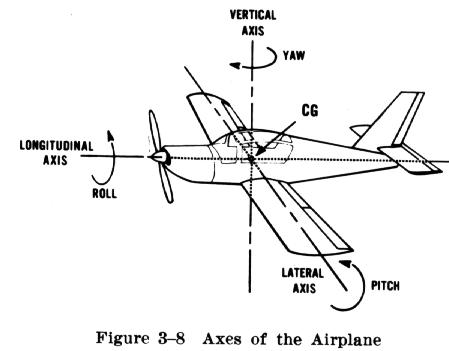# Is transverse synonym of lateral?

Longitudinal axis (x) is the axis orthogonal to its lateral directions (y and z), but what is transverse direction? Is transverse just another term for lateral?

The terms lateral and transverse often get mixed up, because they can refer to the same thing, although this is not necessary.

To my understanding any lateral is transverse but the opposite is not necessarily true.

Transverse axis is any axis perpendicular to the longitudinal axis

Lateral axis (to my understanding/how I'd use it) implies being to the side, so in a sense it accounts for gravity. So Lateral axis describes an axis that is perpendicular to the longitudinal and its also perpendicular to the gravity axis (parallel to the horizontal plane).

## 1. Movements of blocks.

### 1.1. Block moving horizontally

for the following example:• The longitudinal axis is along the direction of movement so its parallel to X.
• Y an Z can be both termed transverse axis.
• Z can be termed lateral axis

### 1.2Mass moving vertically

for the following example:• Again The longitudinal axis is along the direction of movement so its parallel to Y.
• X and Z can be both termed transverse axis
• X and Z can be termed lateral axis (this is a singularity)

### 1.3. mass moving on an incline

In the following example• Again the longitudinal axis is along the direction of movement so its none of the XYZ
• Y can be termed transverse axis
• Y can be termed lateral axis

## 2. Forces

With forces -in my personal experience- there is usually less confusion .

• Transverse forces: refer to any force perpendicular to the longitudinal axis
• Lateral forces: refer to any force perpendicular to the longitudinal axis and parallel to horizontal plane

So in the following example:• Transverse forces are: Radial force and lateral force
• Lateral forces are: well... the lateral force
• This definition of lateral (also perpendicular to gravity) matches the aviation definition, for level flight at least 😅 – Jonathan R Swift Jan 20 at 9:35

Analogous, but not synonymous - typically used in reference to human bodies and aircraft respectivelyIf describing a material property such a steel ; lateral would never ,correctly, be substituted for transverse.

1. "Transverse" is quite clear, it is an axis that makes 90 degrees to the reference axis. So, if the reference axis is x-axis, both the y and z axes are "transverse" to the x-axis.

2. "Lateral" has a few different meanings and usages:

a) "The lateral distance...", means the horizontal distance between the referenced axis and another axis in parallel.

b) "in the lateral direction", means in the direction transverse to the longitudinal axis of an object.

c) "The lateral force", means a horizontal force, that is acting in direction transverse to the gravity.